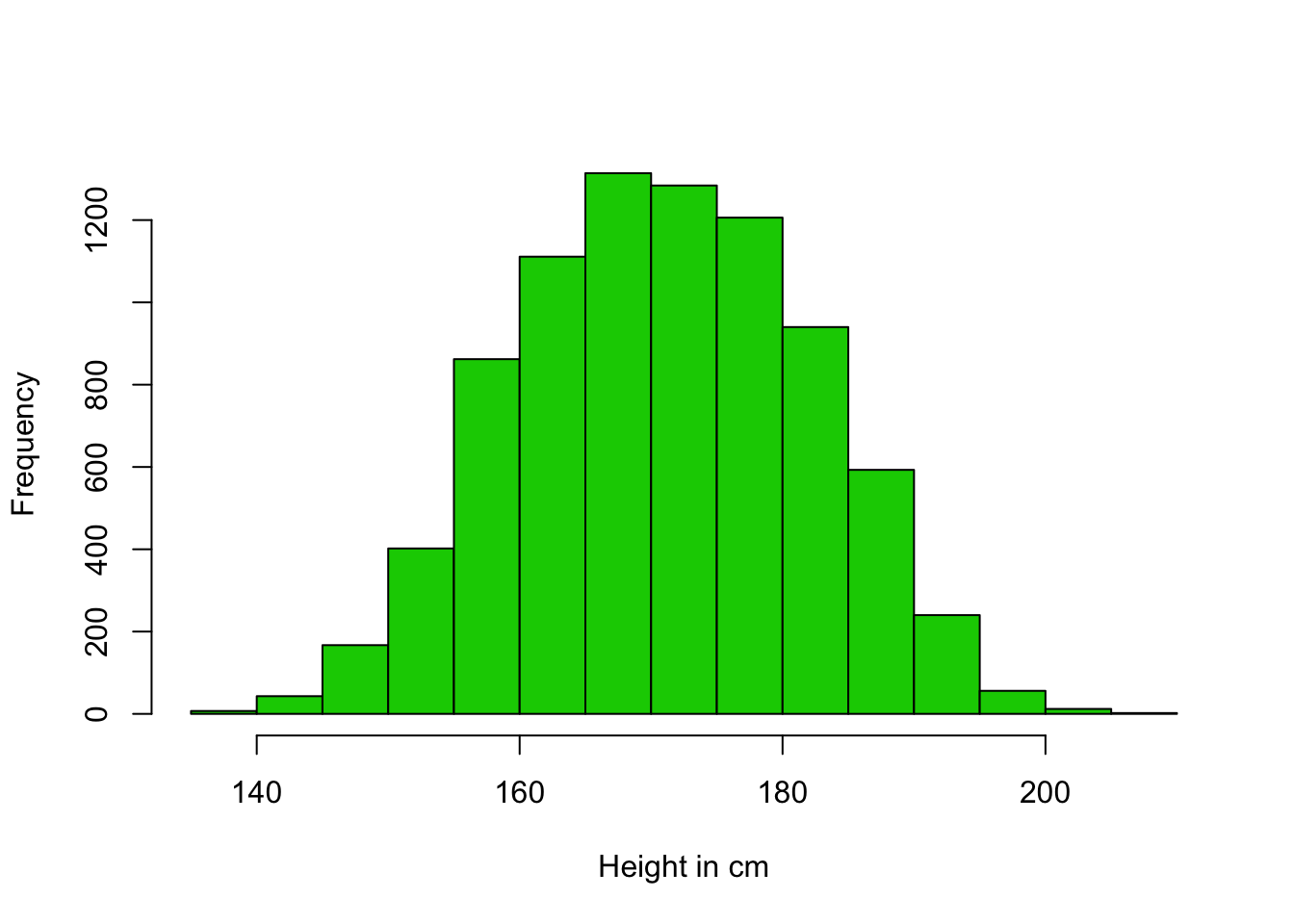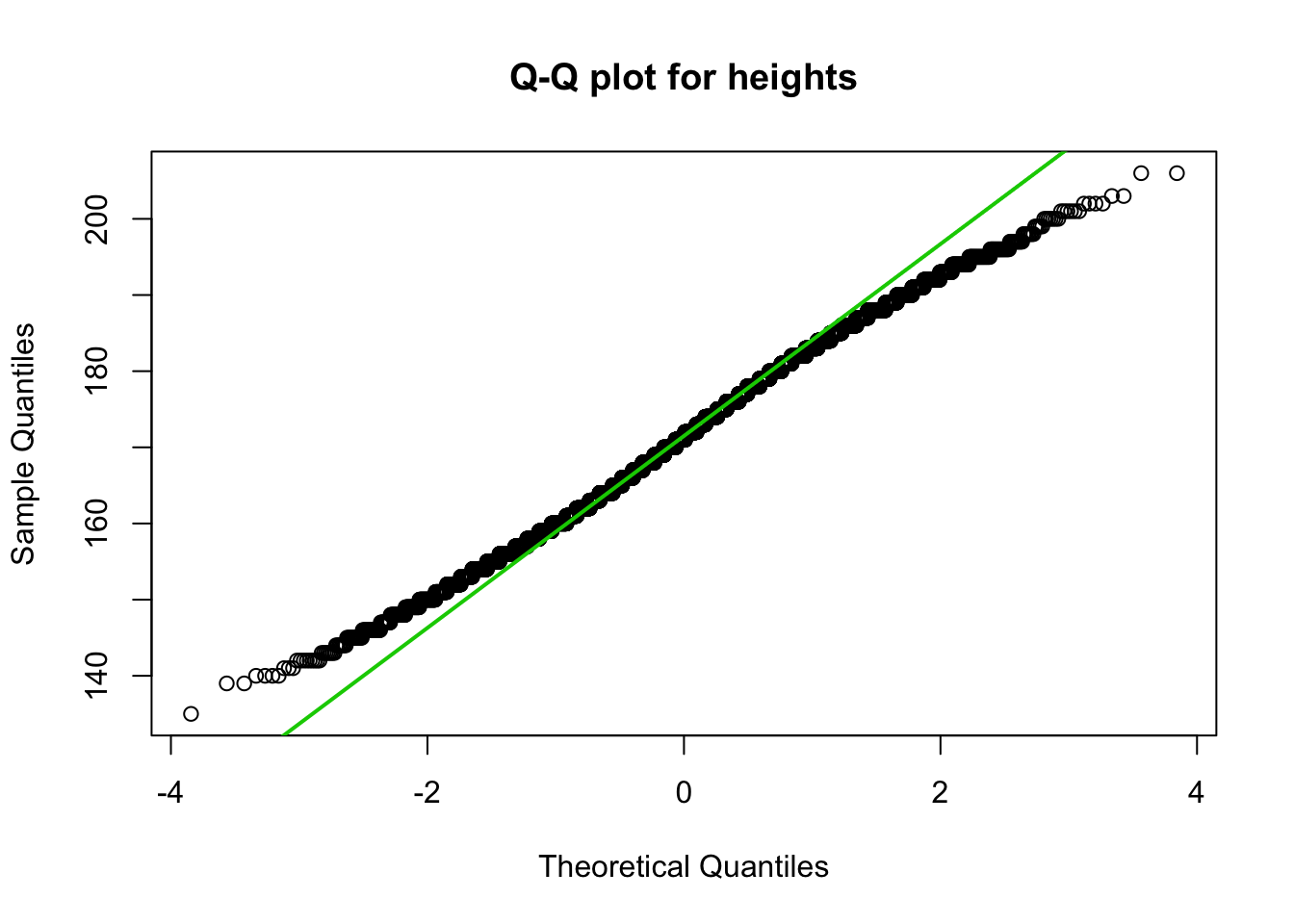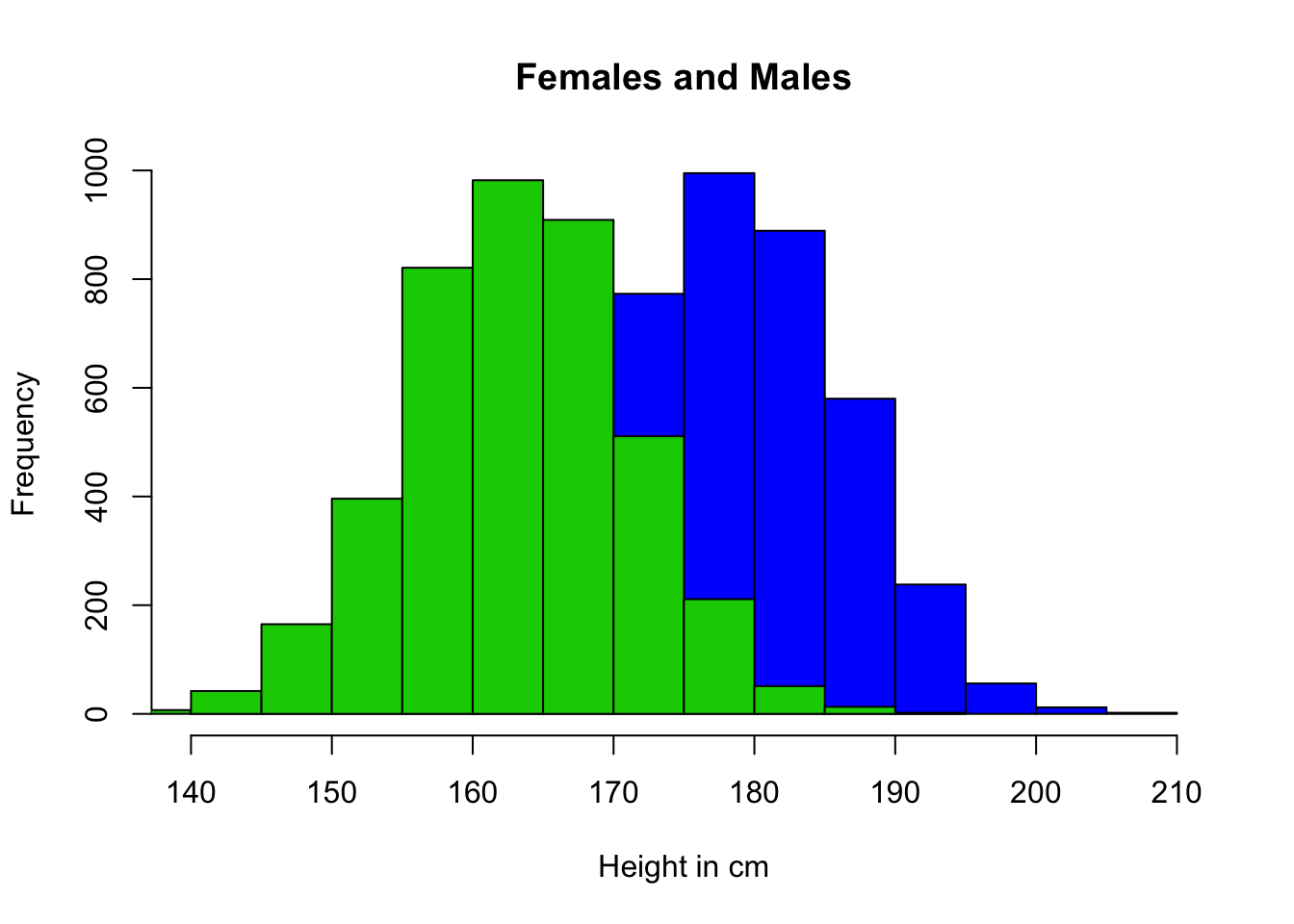#### Data preparation

Now we are ready to do some exercises. Therefore we load the `students` data set. You may download the `students.csv` file here. First, we load the data set and assign a proper name to the data set.

``students <- read.csv("https://userpage.fu-berlin.de/soga/200/2010_data_sets/students.csv")``

The students data set consists of 8239 rows, each of them representing a particular student, and 16 columns, each of them corresponding to a variable/feature related to that particular student. These self-explaining variables are: stud.id, name, gender, age, height, weight, religion, nc.score, semester, major, minor, score1, score2, online.tutorial, graduated, salary. In this sections we use the `height` variable to exercise what we have discussed so far.

First, we want to assure that we are dealing with normally distributed data. If a variable is normally distributed, then, for a large sample, a histogram of the observations should be roughly bell shaped.

``````hist(students\$height,
breaks = 'Sturges',
xlab = 'Height in cm',
main = '',
col = 3)``````By inspecting the plot we may conclude that the `height` variable is normally distributed, however, especially for small samples, ascertaining a clear shape in a histogram and, in particular, whether it is bell shaped is often difficult. Thus, a more sensitive graphical technique is required for assessing normality. Normal probability plots provide such a technique. The idea behind a normal probability plot is simple: Compare the observed values of the variable to the observations expected for a normally distributed variable. More precisely, a normal probability plot is a plot of the observed values of the variable versus the normal scores of the observations expected for a variable having the standard normal distribution. If the variable is normally distributed, the normal probability plot should be roughly linear (i.e., fall roughly in a straight line) (Weiss 2010).

When using a normal probability plot to assess the normality of a variable, we must remember two things:

1. The decision of whether a normal probability plot is roughly linear is a subjective one, and
2. that we are using only a limited number of observations of that particular variable to make a judgment about all possible observations of the variable.

In R we may apply the `qqnorm` and the `qqline` functions for plotting normal probability plots often referred to as Q-Q plots.

``````# Heights
qqnorm(students\$height, main = 'Q-Q plot for heights')
qqline(students\$height, col = 3, lwd = 2)``````By inspecting the plot we see that there is some divergence for the sample quantiles compared to the theoretic quantiles at the lower and upper tails. This fact needs a little more attention! What might be the reason for the departure at the upper and lower tail of the distribution? Any guess?

What about gender? Honestly, it is seems natural that the mean height for males and females differs. Let us plot a histogram of the height of males and females.

``````males <- subset(students, gender=='Male')
females <- subset(students, gender=='Female')

hist(males\$height,
breaks = 'Sturges',
xlab = 'Height in cm',
main = 'Females and Males',
col = 4)

hist(females\$height,
breaks = 'Sturges',
col = 3,``````qqnorm(females\$height, main = 'Q-Q plot for the height of female students')